Modern Physics

# Photoelectric effect Definition and Equation

In this post, You’ll Learn About the Photoelectric effect Equation and Much more.
So, if you want to get benefits from this post, you’ll love this Post.
Contents:

• Photoelectric Effect Definition
• Photoelectric Formula
• Applications of the Photoelectric Effect

## What is the Photoelectric Effect?

“When the light of suitable frequency falls on a metal surface, electrons are emitted from the metal surface. This phenomenon is called the photoelectric effect.”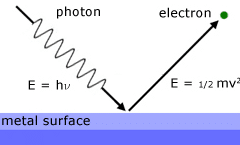The electron emitted in this process is known as photoelectrons.
Explanation
Experimental Setup for this Effect:
The apparatus used to observe this effect is shown in the above Figure: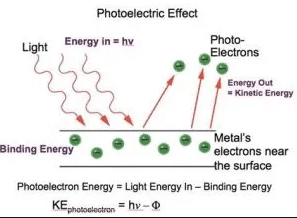It consists of an evacuated glass tube in which two electrodes are scaled. The electrode ‘C’ which is connected to the negative terminal of the battery is called an emitter while the electrode ‘A’ which is connected to the positive terminal of the battery is called a collector.
When the light of frequency ‘u’ is allowed to an incident on the emitter ‘C’ photoelectrons are emitted. If a suitable potential difference is set up between emitter ‘C’ and collector ‘A’ then photoelectrons are attracted by the collector ‘A’ and a current start to flow. This current is known as photoelectric current, measured by an ammeter.
The graph between V and iIf a graph is plotted between the potential difference V and the photoelectric current I then curve obtained. This curve shows that if V is positive and large enough, the photoelectric current reaches a constant saturation value at which all the electrons which are ejected by the emitter ‘E’ are collected by the collector ‘C’.
If we reduce ‘V’ to zero, the photoelectric current does not immediately drop to zero. The reason is that the electrons that are emitted from the emitter still have some speed. So they reach a collector and cause the current to flow.

### Maximum Energy Of Photoelectrons

The maximum energy of photoelectric can be determined by reversing the connection of the battery. Now the emitter ‘E’ will be at positive potential while collector ‘C’ will be at a negative potential. In this condition collector, ‘C’ is at negative potential and will repel photoelectrons.
If the emitter is made more and more negative, then at a certain value of potential, no photoelectrons will reach collector ‘C’. This potential is called the stopping potential. At stopping potential photoelectric current drops to zero.
If Vº is the stopping potential, then this potential difference multiplied by the electric charge gives the kinetic energy of the most energetic photoelectrons.Here v max is the maximum speed of the most energetic photoelectrons.
The graph between stopping potential Vº  and frequency ν: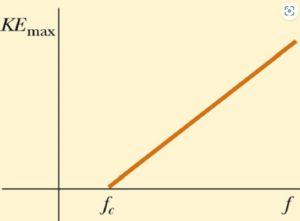If a graph is plotted between the stopping potential V° and the frequency ‘u’ of the incident photon, we get a straight line as shown in the above figure. The line cuts the frequency axis at a point V°, where stopping potential is zero. The frequency V°  is called cut off frequency, or threshold frequency.

### Threshold Frequency equation

It is stated as “The minimum frequency of incident light to produce a photoelectric effect is called threshold frequency or cut-off frequency.”
The light having a frequency less than the threshold frequency cannot photoelectrons from the metal surface, so no photoelectric effect is observed.

### Result Observed During the Photoelectric Effect

The following results are observed during the effect:

1. No photoelectrons are emitted if the frequency of incident light is less than the threshold frequency V° even if the intensity of light is very large.
2. For different materials, threshold frequency is different i.e. threshold frequency depends upon the nature of the material.
3. The number of photoelectrons emitted is directly proportional to the intensity of the incident light.
4. The K.E. of photoelectrons depends upon the frequency of incident light.
5. The K.E. of photoelectrons does not depend upon the intensity of light.
6. The photoelectrons are emitted even when the weakest beam of light (less intense beam) having a frequency greater than the threshold frequency falls on the metal surface.

#### Failure of Classical Wave Theory of Light

The classical wave theory of light could not explain the following three major features of the photoelectric effect.

• The Intensity Problem: wave theory requires that the oscillating electric vector E of the light wave increases in amplitude as the intensity of the light beam is increased. Since the force applied to the electron is F =e E, this suggests that the K.E. of the photoelectrons should increase as the light beam is made more intense. But the experimental results show that the K.E. of photoelectrons is independent of the intensity of the light.
• The Frequency Problem: According to wave theory, the photoelectric effect should occur for any frequency of the light provides that the light is intense enough to supply the energy required to eject the photoelectrons. However, the experimental results show that there exists a cut-off frequency for each surface. For the frequencies less than cut-off frequency u° the photoelectric effect does not occur, no matter how intense light is used.
• The Time Delay Problem: In classical wave theory, light energy is uniformly distributed over the wavefront. Thus if the light is feeble enough, there should be a measurable time lag between the incidence of the light and the ejection of the photoelectrons. During this interval, the electron should be absorbing energy from the beam until it escapes out of the surface. However, no detectable time delay has ever been measured.

### Who Found Photoelectric Effect?

According to Plank’s quantum theory, the emission of light energy is not continuous. Light energy is emitted in the form of bundles called photons. The energy of each photon is given by:

E=hν

Where ‘h’ is Plank’s constant and ‘ν’ is the frequency of the photon. This relation shows that the energy of the photon depends upon frequency.

In 1905, Einstein found the photoelectric effect on the basis of quantum theory. According to Einstein, when a photon of energy hν falls on the metal surface it transfers all its energy to the electron of the metal and the electron is emitted out from the metal surface. This phenomenon is called the photoelectric effect.

If the energy of the photon ‘hν’ is less than the work function hν, then no emission of electrons takes place.

## Photoelectric effect equation

The minimum amount of energy required by an electron to eject from the metal surface is called work function. It is denoted by Φ, thus
Work function formula
Φ=hν0
Where νis the threshold frequency.
When a photon of energy hν falls on a metal surface, it transfers all its kinetic energy to the electron of the metal. A part of this energy is used as a work function to eject the electron from the metal surface and the remaining energy will appear as K.E of the electron: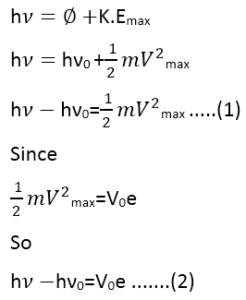Equation (1) and (2) represents Einstein’s photoelectric Equations.

#### Einstein’s photon  theory meets the three objections raised against the wave theory

Einstein’s photon theory also meets the three objections raised the wave theory for the interpretation of the photoelectric effect.

1. ##### The intensity problem

According to Einstein’s photon theory, if the intensity of the incident light is increased, the number of electrons emitted from the metal surface also increases. If we double the intensity of the light, the number of electrons emitted from the metal surface also becomes double, and thus double the photoelectric current. However, the energy of the photoelectrons remains the same.

2. ##### The Intensity problem

The second objection can be met if K.E max=0
Then  Einstein’s photoelectric equation will become: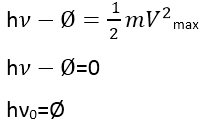Where ν=ν0

⇒K.E max=0 only when ν=ν0

Now if the frequency of the incident light photon is ν < ν0, then hν <φ, so the incident photon will not have enough energy to eject the photoelectrons, no matter how intense light is used.

##### 3.Time delay problem

The third objection “Time delay problem” can also be met using photon theory. As the energy supplied to the electrons is in concentrated bundles. It is not spread uniformly over the wavefronts as was the case in the wave theory of light. So when a photon strikes with the electrons, it transfers all of its energy to the electron without any time lag.

### Related Articles

1. Tage Elkjær Larsen says:

You are reat and are able to explayn it.Difficult stof,but you are so dear clever.Thank you very much

2. nike dunk sb says:

Pretty! This was a really wonderful article. Many thanks for providing this information.

3. A fascinating discussion is worth comment. There’s no doubt